# Data Structures for PHP Devs: Graphs

PHP

Share:

#### Free JavaScript Book!Write powerful, clean and maintainable JavaScript.

RRP \$11.95

In one of my previous articles I introduced you to the tree data structure. Now I’d like to explore a related structure – the graph. Graphs have a number of real-world applications, such as network optimization, traffic routing, and social network analysis. Google’s PageRank, Facebook’s Graph Search, and Amazon’s and NetFlix’s recommendations are some examples of graph-driven applications.

In this article I’ll explore two common problems in which graphs are used – the Least Number of Hops and Shortest-Path problems.

A graph is a mathematical construct used to model the relationships between key/value pairs. A graph comprises a set of vertices (nodes) and an arbitrary number of edges (lines) which connect them. These edges can be directed or undirected. A directed edge is simply an edge between two vertices, and edge A→B is not considered the same as B→A. An undirected edge has no orientation or direction; edge A-B is equivalent to B-A. A tree structure which we learned about last time can be considered a type of undirected graph, where each vertex is connected to at least one other vertex by a simple path.

Graphs can also be weighted or unweighted. A weighted graph, or a network, is one in which a weight or cost value is assigned to each of its edges. Weighted graphs are commonly used in determining the most optimal path, most expedient, or the lowest “cost” path between two points. GoogleMap’s driving directions is an example that uses weighted graphs.

## The Least Number of Hops

A common application of graph theory is finding the least number of hops between any two nodes. As with trees, graphs can be traversed in one of two ways: depth-first or breadth-first. We covered depth-first search in the previous article, so let’s take a look at breadth-first search.

Consider the following graph: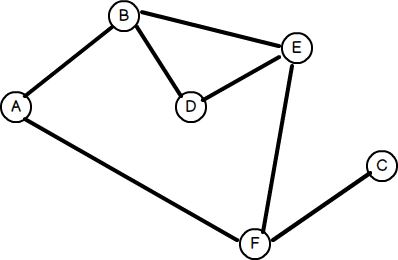For the sake of simplicity, let’s assume that the graph is undirected – that is, the edges in any direction are identical. Our task is to find the least number of hops between any two nodes.

In a breadth-first search, we start at the root node (or any node designated as the root), and work our way down the tree level by level. In order to do that, we need a queue to maintain a list of unvisited nodes so that we can backtrack and process them after each level.

The general algorithm looks like this:

```1. Create a queue
2. Enqueue the root node and mark it as visited
3. While the queue is not empty do:
3a. dequeue the current node
3b. if the current node is the one we're looking for then stop
3c. else enqueue each unvisited adjacent node and mark as visited
```

But how do we know which nodes are adjacent, let alone unvisited, without traversing the graph first? This brings us to the problem of how a graph data structure can be modelled.

### Representing the Graph

There are generally two ways to represent a graph: either as an adjacency matrix or an adjacency list. The above graph represented as an adjacency list looks like this: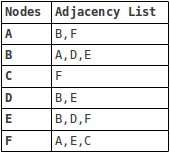Represented as a matrix, the graph looks like this, where 1 indicates an “incidence” of an edge between 2 vertices: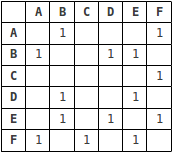Adjacency lists are more space-efficient, particularly for sparse graphs in which most pairs of vertices are unconnected, while adjacency matrices facilitate quicker lookups. Ultimately, the choice of representation will depend on what type of graph operations are likely to be required.

Let’s use an adjacency list to represent the graph:

``````<?php
\$graph = array(
'A' => array('B', 'F'),
'B' => array('A', 'D', 'E'),
'C' => array('F'),
'D' => array('B', 'E'),
'E' => array('B', 'D', 'F'),
'F' => array('A', 'E', 'C'),
);``````

And now, let’s see what the general breadth-first search algorithm’s implementation looks like:

``````<?php
class Graph
{
protected \$graph;
protected \$visited = array();

public function __construct(\$graph) {
\$this->graph = \$graph;
}

// find least number of hops (edges) between 2 nodes
// (vertices)
// mark all nodes as unvisited
foreach (\$this->graph as \$vertex => \$adj) {
\$this->visited[\$vertex] = false;
}

// create an empty queue
\$q = new SplQueue();

// enqueue the origin vertex and mark as visited
\$q->enqueue(\$origin);
\$this->visited[\$origin] = true;

// this is used to track the path back from each node
\$path = array();
\$path[\$origin]->setIteratorMode(
);

\$path[\$origin]->push(\$origin);

\$found = false;
while (!\$q->isEmpty() && \$q->bottom() != \$destination) {
\$t = \$q->dequeue();

if (!empty(\$this->graph[\$t])) {
foreach (\$this->graph[\$t] as \$vertex) {
if (!\$this->visited[\$vertex]) {
// if not yet visited, enqueue vertex and mark
// as visited
\$q->enqueue(\$vertex);
\$this->visited[\$vertex] = true;
// add vertex to current path
\$path[\$vertex] = clone \$path[\$t];
\$path[\$vertex]->push(\$vertex);
}
}
}
}

if (isset(\$path[\$destination])) {
echo "\$origin to \$destination in ",
count(\$path[\$destination]) - 1,
" hopsn";
\$sep = '';
foreach (\$path[\$destination] as \$vertex) {
echo \$sep, \$vertex;
\$sep = '->';
}
echo "n";
}
else {
echo "No route from \$origin to \$destinationn";
}
}
}``````

Running the following examples, we get:

``````<?php
\$g = new Graph(\$graph);

// least number of hops between D and C
// outputs:
// D to C in 3 hops
// D->E->F->C

// least number of hops between B and F
// outputs:
// B to F in 2 hops
// B->A->F

// least number of hops between A and C
// outputs:
// A to C in 2 hops
// A->F->C

// least number of hops between A and G
// outputs:
// No route from A to G``````

If we had used a stack instead of a queue, the traversal becomes a depth-first search.

## Finding the Shortest-Path

Another common problem is finding the most optimal path between any two nodes. Earlier I mentioned GoogleMap’s driving directions as an example of this. Other applications include planning travel itineraries, road traffic management, and train/bus scheduling.

One of the most famous algorithms to address this problem was invented in 1959 by a 29 year-old computer scientist by the name of Edsger W. Dijkstra. In general terms, Dijkstra’s solution involves examining each edge between all possible pairs of vertices starting from the source node and maintaining an updated set of vertices with the shortest total distance until the target node is reached, or not reached, whichever the case may be.

There are several ways to implement the solution, and indeed, over years following 1959 many enhancements – using MinHeaps, PriorityQueues, and Fibonacci Heaps – were made to Dijkstra’s original algorithm. Some improved performance, while others were designed to address shortcomings in Dijkstra’s solution since it only worked with positive weighted graphs (where the weights are positive values).

Here’s an example of a (positive) weighted graph: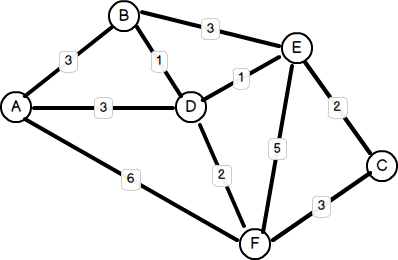We can represent this graph as an adjacency list, as follows:

``````<?php
\$graph = array(
'A' => array('B' => 3, 'D' => 3, 'F' => 6),
'B' => array('A' => 3, 'D' => 1, 'E' => 3),
'C' => array('E' => 2, 'F' => 3),
'D' => array('A' => 3, 'B' => 1, 'E' => 1, 'F' => 2),
'E' => array('B' => 3, 'C' => 2, 'D' => 1, 'F' => 5),
'F' => array('A' => 6, 'C' => 3, 'D' => 2, 'E' => 5),
);``````

And here’s an implementation using a PriorityQueue to maintain a list of all “unoptimized” vertices:

``````<?php
class Dijkstra
{
protected \$graph;

public function __construct(\$graph) {
\$this->graph = \$graph;
}

public function shortestPath(\$source, \$target) {
// array of best estimates of shortest path to each
// vertex
\$d = array();
// array of predecessors for each vertex
\$pi = array();
// queue of all unoptimized vertices
\$Q = new SplPriorityQueue();

foreach (\$this->graph as \$v => \$adj) {
\$d[\$v] = INF; // set initial distance to "infinity"
\$pi[\$v] = null; // no known predecessors yet
foreach (\$adj as \$w => \$cost) {
// use the edge cost as the priority
\$Q->insert(\$w, \$cost);
}
}

// initial distance at source is 0
\$d[\$source] = 0;

while (!\$Q->isEmpty()) {
// extract min cost
\$u = \$Q->extract();
if (!empty(\$this->graph[\$u])) {
foreach (\$this->graph[\$u] as \$v => \$cost) {
// alternate route length to adjacent neighbor
\$alt = \$d[\$u] + \$cost;
// if alternate route is shorter
if (\$alt < \$d[\$v]) {
\$d[\$v] = \$alt; // update minimum length to vertex
\$pi[\$v] = \$u;  // add neighbor to predecessors
//  for vertex
}
}
}
}

// we can now find the shortest path using reverse
// iteration
\$S = new SplStack(); // shortest path with a stack
\$u = \$target;
\$dist = 0;
// traverse from target to source
while (isset(\$pi[\$u]) && \$pi[\$u]) {
\$S->push(\$u);
\$dist += \$this->graph[\$u][\$pi[\$u]]; // add distance to predecessor
\$u = \$pi[\$u];
}

// stack will be empty if there is no route back
if (\$S->isEmpty()) {
echo "No route from \$source to \$targetn";
}
else {
// add the source node and print the path in reverse
// (LIFO) order
\$S->push(\$source);
echo "\$dist:";
\$sep = '';
foreach (\$S as \$v) {
echo \$sep, \$v;
\$sep = '->';
}
echo "n";
}
}
}``````

As you can see, Dijkstra’s solution is simply a variation of the breadth-first search!

Running the following examples yields the following results:

``````<?php
\$g = new Dijkstra(\$graph);

\$g->shortestPath('D', 'C');  // 3:D->E->C
\$g->shortestPath('C', 'A');  // 6:C->E->D->A
\$g->shortestPath('B', 'F');  // 3:B->D->F
\$g->shortestPath('F', 'A');  // 5:F->D->A
\$g->shortestPath('A', 'G');  // No route from A to G``````

## Summary

In this article I’ve introduced the basics of graph theory, two ways of representing graphs, and two fundamental problems in the application of graph theory. I’ve shown you how a breadth-first search is used to find the least number of hops between any two nodes, and how Dijkstra’s solution is used to find the shortest-path between any two nodes.

Image via Fotolia### Ignatius Teo

Ignatius Teo is a freelance PHP programmer and has been developing n-tier web applications since 1998. In his spare time he enjoys dabbling with Ruby, martial arts, and playing Urban Terror.

#### New books out now!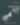Learn how Git works, and how to use it to streamline your workflow!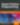Google, Netflix and ILM are Python users. Maybe you should too?